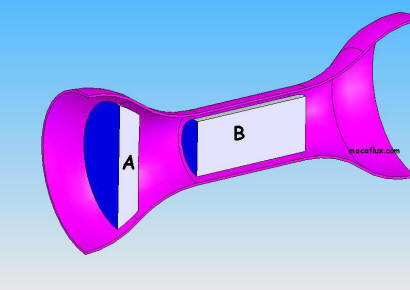The flow continuity equation :

Section A x Speed ​​A = Section B x Speed ​​B = constant volume flow

• we deduce the velocity at point B
Speed ​​B = Section A / Section B x Speed ​​A• We estimated in formulas continuity flow volume above, the fluid is incompressible. For a compressible fluid continuity equation must be applied to the mass flow rate:

Section A x Speed A= Section B xSpeed B = mass flow constant

• The equation of continuity applied to constant mass flow gives us:

Density A x Volume A x Section A = Density B x Volume B x SectionB

For all fumulas aboves:

• Density in kg/m3
• volume in m3
• section in m²
• spedd in m/sec
• volume flow in m3/sec
• mass flow in kg/sec
When section decreases, the flow remains constant, the fluid accelerates, the pressure decreases...See Bernoulli and Venturi